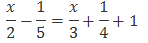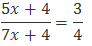# Linear equations in One variable Class 8 Notes

In this page we will explain the topics for the chapter 8 of Linear equations in One variable Class 8 Maths.We have given quality Linear equations in One variable Class 8 Notes and video to explain various things so that students can benefits from it and learn maths in a fun and easy manner, Hope you like them and do not forget to like , social share and comment at the end of the page.
Table of Content

## What is algebraic expression

Algebraic expression is the expression having constants and variable. It can have multiple variable and multiple power of the variable
Example
$10x$
$2x -3$
$3x + y$
$2xy + 11$
$x^2 +2$

## What is algebraic equation

We already know the below terms from previous class
What is equation
An equation is a condition on a variable.
What is variable
A variable takes on different numerical values; its value is not fixed. Variables are denoted usually by letters of the alphabets, such as x, y, z, l, m, n, p, etc

An algebraic equation is an equality involving variables. It says that the value of the expression on one side of the equality sign is equal to the value of the expression on the other side.
Example
$5x = 25$
$2x - 3 = 9$
$11xy+1 =98$
$x^2 +1=y^2$
Important points to remember
1. These all above equation contains the equality (=) sign.
2. The above equation can have more than one variable
3. The above equation can have highest power of variable > 1
4. The expression on the left of the equality sign is the Left Hand Side (LHS). The expression on the right of the equality sign is the Right Hand Side (RHS)
5. In an equation the values of the expressions on the LHS and RHS are equal. This happens to be true only for certain values of the variable. These values are the solutions of the equation.
6. We assume that the two sides of the equation are balanced. We perform the same mathematical operations on both sides of the equation, so that the balance is not disturbed. We get the solution after generally performing few steps

## What is Linear equation in one Variable

We will restrict the above equation with two conditions
a) algebraic equation in one variable
b) variable will have power 1 only
Example
$5x = 25$
$2x - 3 = 9$
We will focus on Linear equation in One variable in this chapter.

## Solving Equations which have Linear Expressions on one Side and Numbers on the other Side

$2x -3= 5$
$3x-11= 22$
How to solve Linear equation in one Variable
1. Transpose (changing the side of the number)  the Numbers to the side where all number are present.  We know the sign of the number changes when we transpose it to other side
2. Now you will have a equation have variable on one side and number on other side. Add/subtract on  both the side to get single term
3. Now divide or multiply on both the side to get the value of the variable
Example
$2x -3= 5$
Solution
Transposing 3 to other side
$2x=5+3$
$2x=8$
Dividing both the sides by 2
x=4
Watch this tutorial on how to solve Equations which have Linear Expressions on one Side and Numbers on the other Side

## Word Problem on Simple Linear equation in one variable

Linear equation can be used to solve many word Problem. The procedure is simple
1. First read the problem carefully. Write down the unknown and known
2. Assume one of the unknown to x  and find the other unknown in term of that
3. Create the linear equation based on the condition given
4. Solve them by using the above method
Example:
The perimeter of a rectangular swimming pool is 154 m. Its length is 2 m more than twice its breadth. What are the length and the breadth of the pool?
Solution
The unknown are length and breadth.
Let the breadth be x m.
Then as per question the length will be (2x + 2) m.
Perimeter of swimming pool = 2(l + b) = 154 m
$2(2x + 2 + x) = 154$
$2(3x + 2) = 154$
Dividing both sides by 2
$3x + 2 = 77$
Transposing 2 to R.H.S, we obtain
$3x = 77 - 2$
$3x = 75$
Dividing 3 on both the sides
$x = 25$
So
Breath is 25 m
Length =2x + 2 = 2 × 25 + 2 = 52m
Hence, the breadth and length of the pool are 25 m and 52 m respectively.
Watch this tutorial on Word Problem on Simple Linear equation in one variable

## Solving Equations having the Variable on both Sides

$2x -3= 6-x$
$3x-11= 4x$
How to solve them
1. Here we Transpose (changing the side of the number) both the variable and  Numbers to the side so that one side contains only the number and other side contains only the variable. We know the sign of the number changes when we transpose it to other side.Same is the case with Variable
2. Now you will have a equation have variable on one side and number on other side. Add/subtract on  both the side to get single term
3. Now divide or multiply on both the side to get the value of the variable
Example
$2x -3= 6-x$
Solution
Transposing 3 to RHS and x to LHS
$2x+x=6+3$
$3x=9$
Dividing both the sides by 3
$x=3$
Watch this tutorial on Solving Equations having the Variable on both Sides

## Reducing Equations to Simpler Form

You will find many situations where the linear equation may be having number in denominator. We can perform the below steps to simplify them and solve it
1. Take the LCM of the denominator of both the LHS and RHS
2. Multiple the LCM on both the sides, this will reduce the number without denominator and we can solve using the method described above
Example
Solve the linear equationSolutionL.C.M. of the denominators, 2, 3, 4, and 5, is 60.
So Multiplying both sides by 60, we obtain
$30x - 12 = 20x + 15 +60$
Transposing 20x  to R.H.S and 12 to L.H.S, we obtain
$30x- 20x = 15 + 12+60$
$10x= 87$
Dividing both the sides by 10
$x=\frac {[87}{10}=8.7$
Watch this tutorial on Reducing Equations to Simpler Form

## Equations Reducible to the Linear Form

Let’s explain this with an example
The ages of Hari and Harry are in the ratio 5:7. Four years from now the ratio of their ages will be 3:4. Find their present ages.
Solution
Let the common ratio between their ages be x. Therefore, Hari’s age and Harry’s age will be 5x years and 7x years respectively and four years later, their ages will be (5x + 4) years and (7x + 4) years respectively.
According to the situation given in the question,Now this is not a linear equation as we studied, but if you observe, we can reduce to linear equation.
We multiply both the sides by (7x+4)
Multiplying both the sides by (7x+4)
$(5x+4) =\frac {3(7x+4)}{4}$
or
$20x+16=21x+12$
Transposing 20x to RHS and 12 to LHS
4=x
x=4
Hari’s age = 5x years = (5 × 4) years = 20 years
Harry’s age = 7x years = (7 × 4) years = 28 years
Therefore, Hari’s age and Harry’s age are 20 years and 28 years respectively.

Watch this tutorial on how to solve word problem in linear equation

## Practice Questions

1. $6 x + 3 = 3 x + 4$
2. $11 x + 4 = 4 x + 10$
3. $13 x + 1 = x + 9$
4. $3 x + 1 = x + 3$
5. $15 x + 5 = 5 x + 5$
6. $12 x + 9 = 9 x + 7$
7. $7 x + 1 = x + 4$
8. $19 x + 10 = 10 x + 4$
9. $5 x + 4 = 4 x + 6$
10. $9 x + 5 = 5 x + 4$
11. $11 x + 1 = x + 8$
12. $14 x + 10 = 10 x + 6$

## Frequently asked Questions on CBSE Class 8 Maths Chapter 2: Linear equations in One Variable

### What is LHS and RHS Class 8??

In an equation ,The expression on the left of the equality sign is the Left Hand Side (LHS). The expression on the right of the equality sign is the Right Hand Side (RHS)

### What is the General Form of a linear equation in One Variable?

The general form of the linear equations in one variable is Ax + B = 0 where x is the variable and A is the coefficient of x and b is the constant term. Example 2x +3=0

### What are the properties of linear equation?

Properties of Linear equation (i) Variable will have Power 1 only (ii) It cannot be denominator of the fraction

## Summary

Here is Linear equations in One variable Class 8 notes Summary
1. Linear equations in One Variable is an algebriac equation where we have one variable and it has power 1 only.
2. The solution of the linear equation can be integers or fractions
3. We can transpose both the constant and linear expression in the linear equations to solve the equation